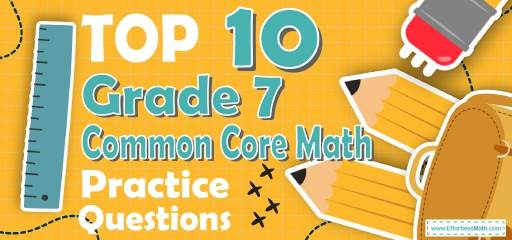# Top 10 7th Grade Common Core Math Practice QuestionsSearching for sample 7th Grade Common Core Math questions and exercises? The best way to help your students prepare for the 7th Grade Common Core Math test is to work through as many Common Core Math practice questions as possible. Here are the top 10 7th Grade Common Core Math practice questions to help students review the most important 7th Grade Common Core Math concepts. These 7th GradeCommon Core Math practice questions are designed to cover mathematics concepts and topics that are found on the actual test. The questions have been fully updated to reflect the latest 2021 7th Grade Common Core guidelines. Answers and full explanations are provided at the end of the post.

## 7th Grade Common Core Math Practice Questions

1- A rope weighs 600 grams per meter of length. What is the weight in kilograms of 12.2 meters of this rope? (1 kilograms = 1000 grams)

A. 0.0732

B. 0.732

C. 7.32

D. 7,320

2- Jason needs an $$75\%$$ average in his writing class to pass. On his first 4 exams, he earned scores of $$68\%, 72\%, 85\%$$, and $$90\%$$. What is the minimum score Jason can earn on his fifth and final test to pass? ___________

3- The following trapezoid are similar. What is the value of $$x$$?

A. 7

B. 8

C. 18

D. 45

4- Right triangle ABC has two legs of lengths 6 cm (AB) and 8 cm (AC). What is the length of the third side (BC)?

A.  4 cm

B. 6 cm

C. 8 cm

D. 10 cm

5- The marked price of a computer is D dollar. Its price decreased by $$20\%$$ in January and later increased by $$10\%$$ in February. What is the final price of the computer in D dollar?

A. 0.80 D

B. 0.88 D

C. 0.90 D

D. 1.20 D

6- $$[6 × (–24) + 8] – (–4) + [4 × 5] ÷ 2 =$$?

A. $$-122$$

B. $$-112$$

C. $$-102$$

D. $$-92$$

7- The area of a circle is $$64 π$$. What is the circumference of the circle?

A. $$8 π$$

B. $$16 π$$

C. $$32 π$$

D. $$64 π$$

8- A $40 shirt now selling for$28 is discounted by what percent?

A. $$20\%$$

B. $$30\%$$

C. $$40\%$$

D. $$60\%$$

9- From last year, the price of gasoline has increased from $1.25 per gallon to$1.75 per gallon. The new price is what percent of the original price?

A. $$72\%$$

B. $$120\%$$

C. $$140\%$$

D. $$160\%$$

10- If $$40\%$$ of a class are girls, and $$25\%$$ of girls play tennis, what fraction of the class play tennis?

A. $$10\%$$

B. $$15\%$$

C. $$20\%$$

D. $$40\%$$

## Best 7th Grade Common Core Math Workbook Resource for 2021

1- C
The weight of 12.2 meters of this rope is: 12.2 $$×$$ 600 g = 7320 g
1 kg = 1000 g therefore,
7320 g $$÷$$ 1000 = 7.32 kg

2- 60
Jason needs an $$75\%$$ average to pass for five exams. Therefore, the sum of 5 exams must be at lease $$5 × 75 = 375$$
The sum of 4 exams is:
68 + 72 + 85 + 90 = 315
The minimum score Jason can earn on his fifth and final test to pass is:
375 – 315 = 60

3- A
It’s needed to have a ratio to find value of $$x$$.
$$\frac{45}{40}=\frac{2x+4}{16}⇒ 40(2x+4)=45×16 ⇒ x=7$$

4- D
Use Pythagorean Theorem: $$a^2 + b^2 = c^2$$
$$62 + 82 = c^2 ⇒ 100 = c^2 ⇒ c = 10$$

5- B
To find the discount, multiply the number by ($$100\% –$$ rate of discount).
Therefore, for the first discount we get:
(D) ($$100\% – 20\%) =$$ (D) (0.80) = 0.80 D
For increase of $$10%$$:
(0.80 D) ($$100\% + 10\%) =$$ (0.85 D) (1.10) = 0.88 D $$= 88\%$$ of D

6- A
Use PEMDAS (order of operation):
$$[6 × (– 24) + 8] – (– 4) + [4 × 5] ÷ 2 = [– 144 + 8] – (– 4) +  ÷ 2 = [– 144 + 8] – (– 4) + 10 = [– 136] – (– 4) + 10 = [– 136] + 4 + 10 = – 122$$

7- B
Use the formula of areas of circles.
Area $$= πr^2 ⇒ 64 π = πr^2 ⇒ 64 = r^2 ⇒ r = 8$$
Radius of the circle is 8. Now, use the circumference formula:
Circumference $$= 2πr = 2π (8) = 16 π$$

8- B
Use the formula for Percent of Change
$$\frac{New \space Value \ – \ Old \space Value}{Old \space Value}× 100\%$$
$$\frac{28-40}{40}× 100\% = – 30\%$$
(negative sign here means that the new price is less than old price).

9- C

The question is this: 1.75 is what percent of 1.25?
Use percent formula:

part $$= \frac{percent}{100}×$$ whole
$$\frac{percent}{100}× 1.25 ⇒ 1.75 = \frac{percent ×1.25}{100}⇒175 =$$ percent $$×1.25 ⇒$$ percent $$= \frac{175}{1.25}= 140$$

10- A
Let $$x$$ be the amount of students in the class.
$$40\%$$ of $$x =$$ girls
$$25\%$$ of girls = tennis \space player
Input $$40\%$$ of a class instead of girls in second formula. Therefore, $$25\%$$ of $$40\%$$ of a class = tennis player
tennis player $$= 10\%$$

Looking for the best resource to help you succeed on the 7th Grade Common Core Math test?

### What people say about "Top 10 7th Grade Common Core Math Practice Questions - Effortless Math: We Help Students Learn to LOVE Mathematics"?

No one replied yet.

X
27% OFF

Limited time only!

Save Over 27%

SAVE $5 It was$18.99 now it is \$13.99